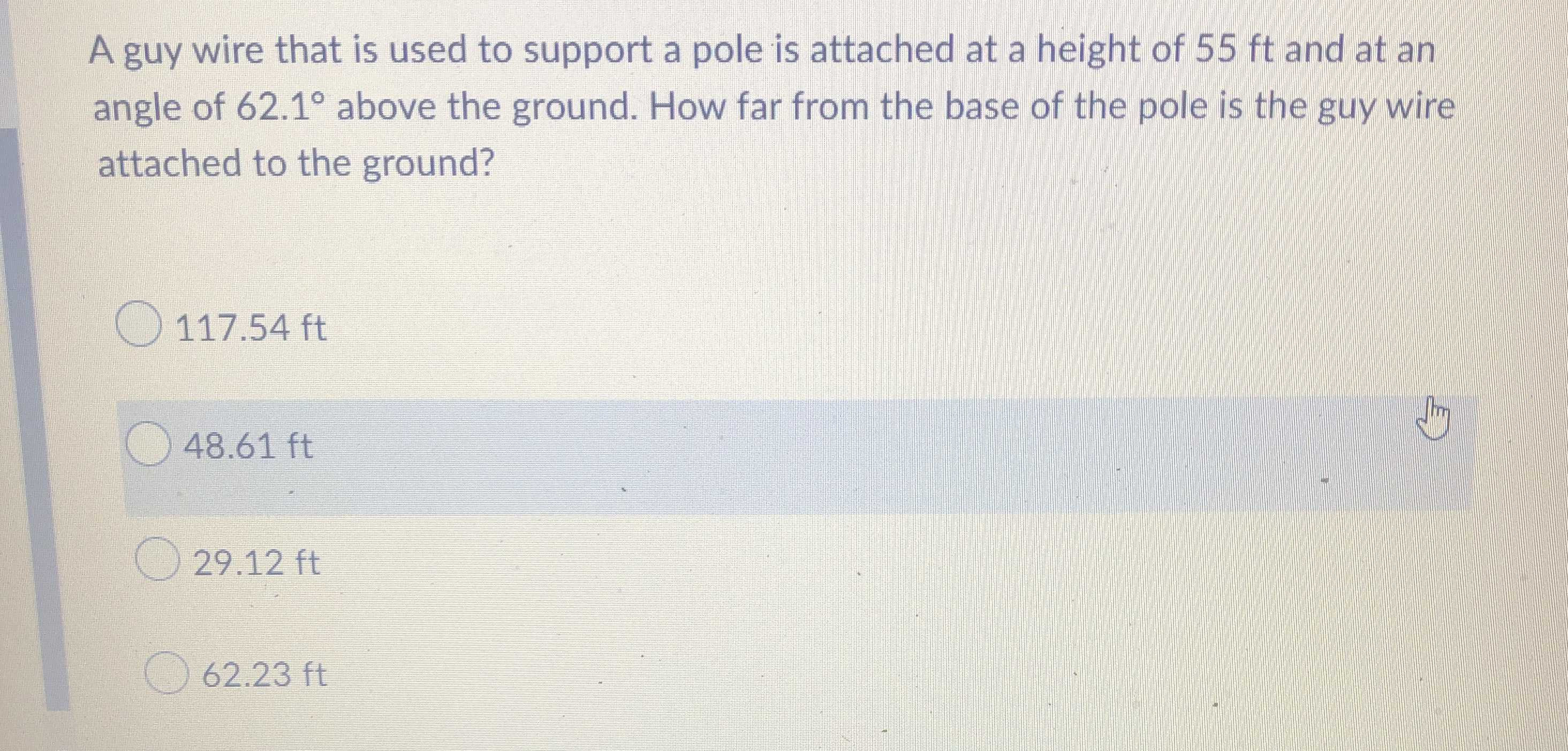### Still have math questions?

Trigonometry
QuestionA guy wire that is used to support a pole is attached at a height of $$55 ft$$ and at an angle of $$62.1 ^ { \circ }$$ above the ground. How far from the base of the pole is the guy wire attached to the ground?

$$117.54 ft$$

$$48.61 ft$$

$$29.12 ft$$

$$62.23 ft$$

$$tan62.1° = \frac{55}{d}$$